### Equilibrium

Static equilibrium

#### Problem:

Two telephone poles are separated by 40 m and connected by a massless wire.  A bird of mass 0.5 kg lands on the wire midway between the poles, causing the wire to sag 2.0 m below horizontal.  What is the tension in the wire?

Solution:

• Concepts:
Static equilibrium
• Reasoning:
All parts of the system are at rest.  Ftotal = τtotal = 0
• Details of the calculation: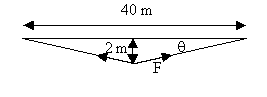2Fy = mg,  Fy = 9.8*0.25 N = 2.45 N.
sinθ = 2/(4 + 400)½.
F = Fy/sinθ = 24.6 N  is the tension in the wire.

#### Problem:

One end of a spring of negligible mass is attached to the ceiling.  When a 250 g mass is placed on the free end (without stretching the spring) and then released, the mass descends 20.0 cm before it changes direction and begins to ascend.  What is the spring constant k (in N/m)?

Solution:

• Concepts:
Hooke's law, equilibrium
• Reasoning
The force exerted by a spring obeys Hooke's law.
• Details of the calculation:
When the mass is hanging on the spring in equilibrium, i.e. when all oscillations have damped out, then the magnitude of the force F = kx from the spring exactly cancels the magnitude of the gravitational force F = mg.
We have mg = kx, or k = mg/x.
m = 0.25 kg is given, we need to know the equilibrium stretch x.
The mass initially oscillates between x = 0 and x = 20 cm.  If we wait long enough the oscillation will damp out and it will come to rest at x = 0.1 m.
k = (0.25kg)(9.8 m/s2)/(0.1 m) = 24.5 N/m.

#### Problem:

Two identical springs with spring constant k = 1 N/m and equilibrium length l = 0.25 m are connected by a middle string of length L = (3/8) m and support a weight w = 0.5 N.
Two strings of length 1 m are loosely connected as shown.
Find the position of the weight below the support and show that it moves up when the middle string is cut.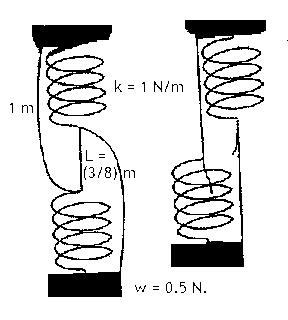Solution:

• Concepts:
Hooke's law: F = -kx, k = spring constant, equilibrium
• Reasoning
Consider two isolated springs with constants k.
Connect them in series.  The effective spring constant is k' = k/2.  (The same force produces twice the displacement.)
Connect them in parallel.  The effective spring constant is k'' = 2k.  (The same force produces half the displacement.)
• Details of the calculation:
Let the length of the unstretched springs be l (m).
The distance from the support to the weight w in case (a) is
d(m) = 2l + 3/8 m + w/k' =  2l + 3/8 m + 1 m = (½  + 3/8 + 1) m  = (15/8) m.
The distance from the support to the weight w in case (b) is
d'(m) = l + 1 m + w/k'' =  (¼ + 1 + ¼) m = (12/8) m
d - d' = (3/8) m,  d > d'.

#### Problem:

Consider a particle moving along the x axis under the influence of the potential

U(x) = ½k(x/a)2 - (1/3)k(x/a)3

where k and a are constants.
(a) Plot the potential.
(b) Find the equilibrium point(s).
(c) Determine whether the equilibrium point(s) are stable or unstable.

Solution:

• Concepts:
Static equilibrium
• Reasoning:
We are asked to find the equilibrium point(s), where F = 0.  An equilibrium point is stable if for small displacements from the equilibrium point the force points towards the equilibrium point.
• Details of the calculation:
(a)  Let k = 1.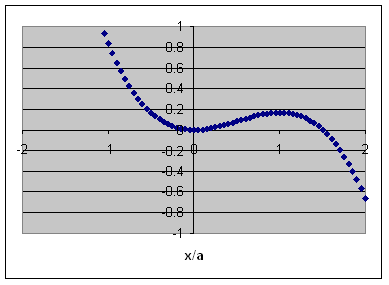(b)  Let x' = x/a.
F = -dU/dx' = -kx' + kx'2  = 0.  x' = x'2,   x/a = 0, 1 are the equilibrium points.
(c)  dF/dx' = -k + 2kx'.
dF/dx'|x'= 0 = -k --> stable equilibrium (restoring force).
dF/dx'|x'= 1 = k -->  unstable equilibrium.

#### Problem:

Two small positively charged spheres are suspended from a common point at the ceiling by the insulating light strings of equal length.  The first sphere has mass m1 and charge q1 while the second one has mass m2 and charge q2.  If the first string makes an angle θ1 with the vertical, find the angle θ2 that the second string makes with the vertical.

Solution:
• Concepts:
Equilibrium
• Reasoning:
In equilibrium the center of mass of the two-pendulum system must lie directly beneath the suspension point.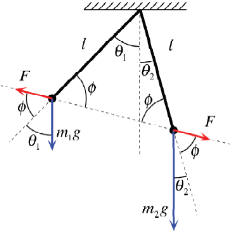• Details of the calculation:
For the center of mass of the two-pendulum system to lie directly beneath the suspension point. We need m1l sinθ1 = m2l sinθ2.
θ2 = sin-1((m1/m2)sinθ1)
Or draw a vector diagram.  Balance the forces.
For example:  m1g sinθ1 = F sinΦ = m2g sinθ2.

Dynamic equilibrium

#### Problem:

A hollow sphere of radius R = 0.5 m rotates about a vertical axis through its center with an angular velocity of ω = 5/s.   Inside the sphere a small block is moving together with the sphere at the height of R/2.   (Let g = 10 m/s2.)
(a)  What is the minimal coefficient of friction to fulfill this condition?
(b)  Find the minimal coefficient of friction for the case of ω  = 8/s.
(c)  Given the minimal coefficient of friction, investigate the problem of stability in both cases,
(i)  for a small change of the position of the block,
(ii)  for a small change of the angular velocity of the sphere.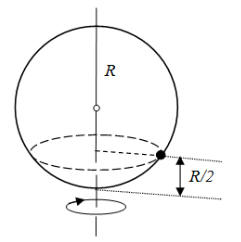Solution:

• Concepts:
Dynamic equilibrium
• Reasoning:
If the block moves along a horizontal circle of radius R sinα, then the net force acting on the block is pointed to the center of this circle.
The vector sum of the normal force exerted by the wall N, the frictional force S, and the weight mg is equal to the centripetal force mω2R sinα.
• Details of the calculation: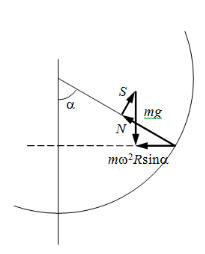(a)  For the horizontal and vertical components we of Fnet we have
2R sinα = N sinα - S cosα,  mg = N cosα + S sinα.
Solving for S and N we obtain
S = mg sinα [1 - ω2 R cosα/g],  N = mg [cosα + ω2 R sin2α/g]
The block does not slip down if  μα = ≥ |S/N| = sinα |1 - ω2 R cosα/g]|/[cosα + ω2 R sin2α/g].
If ω2 R cosα/g < 1 then S points upward, the frictional force prevents the block from sliding down.  This is the case for ω = 5/s.  We have α = 60o and μα min = 0.2259.
(b)  If ω2 R cosα/g >1 then S points downward, the frictional force prevents the block from sliding up.  This is the case for ω = 8/s, and μαmin = 0.1792.
(c)  In case (a) the direction of the frictional force prevents the block from sliding down. μα min increases when α increases by a small amount and decreases when α decreases by a small amount.  If the block is displaced upwards, it slips downward, if it is displaced downward, it stays.  If ω increases be a small amount, the block remains in equilibrium, if ω decreases by a small amount, it slips downwards.
In case (b) the direction of the frictional force prevents the block from sliding up.  μα min increases when α decreases by a small amount and decreases when α increases by a small amount.  If the block is displaced upwards, it stays, if it is displaced downward, it slips upward.  If ω decreases by a small amount, the block remains in equilibrium, if ω increases by a small amount, it slips upward.